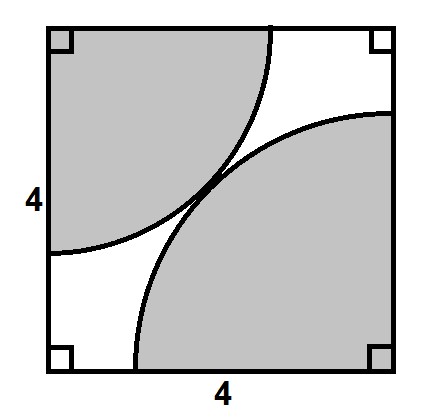# Sectors in a Square

Geometry Level 3Two touching sectors are drawn centred on opposite corners of a square of sidelength 4.

If the total perimeter of the sectors can be expressed in the form $\sqrt{c}(a\pi+b)$ where $a$, $b$ and $c$ are integers and $c$ is a prime, find $a+b+c$.

×